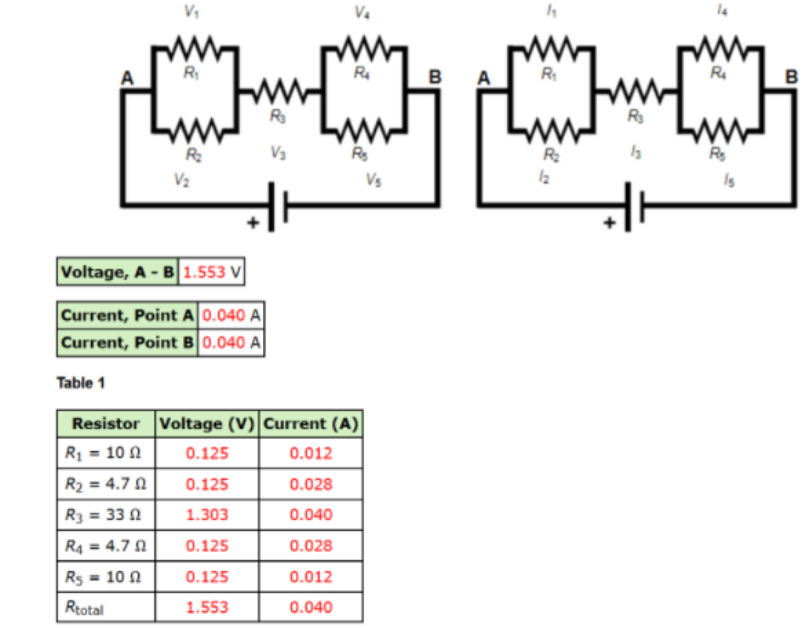# Problem: Measured Data: Kirchhoff's Laws - Voltage and Current in CircuitsThe voltage across and the current through each resistor are shown in the schematic diagram below. 1) Using the schematic of the circuit, calculate the total resistance of the circuit. Req = ?2) Based on the theoretical value of R3 and the voltage measured at R3, calculate the theoretical value of the current l3 using Ohm's Law.Itheoretical = ?3) What do the results suggest about the current coming into a junction and the current leaving a junction? A. The sum of the currents coming into a junction is three times the sum of the currents leaving a junction. B. The sum of the currents coming into a junction is equal to the sum of the currents leaving a junction. C. The sum of the currents coming into a junction is twice the sum of the currents leaving a junction. D. The sum of the currents coming into a junction is half of the sum of the currents leaving a junction. 4) Through any pathway around the circuit, what is the relationship between the total voltage supplied and the voltages along the pathway?

###### FREE Expert Solution

Equivalent resistance for 2 resistors in parallel:

$\overline{){{\mathbf{R}}}_{{\mathbf{eq}}}{\mathbf{=}}\frac{{\mathbf{R}}_{\mathbf{1}}{\mathbf{R}}_{\mathbf{2}}}{{\mathbf{R}}_{\mathbf{1}}\mathbf{+}{\mathbf{R}}_{\mathbf{2}}}}$

85% (190 ratings)###### Problem Details

Measured Data: Kirchhoff's Laws - Voltage and Current in Circuits

The voltage across and the current through each resistor are shown in the schematic diagram below.1) Using the schematic of the circuit, calculate the total resistance of the circuit.

Req = ?

2) Based on the theoretical value of R3 and the voltage measured at R3, calculate the theoretical value of the current l3 using Ohm's Law.

Itheoretical = ?

3) What do the results suggest about the current coming into a junction and the current leaving a junction?

A. The sum of the currents coming into a junction is three times the sum of the currents leaving a junction.

B. The sum of the currents coming into a junction is equal to the sum of the currents leaving a junction.

C. The sum of the currents coming into a junction is twice the sum of the currents leaving a junction.

D. The sum of the currents coming into a junction is half of the sum of the currents leaving a junction.

4) Through any pathway around the circuit, what is the relationship between the total voltage supplied and the voltages along the pathway?